Impact Factor 2.705 | CiteScore 2.2
More on impact ›

# Frontiers in Materials## ORIGINAL RESEARCH article

Front. Mater., 31 January 2019 | https://doi.org/10.3389/fmats.2019.00002

# Optimal Design of the Band Structure for Beam Lattice Metamaterials

• 1IMT School for Advanced Studies Lucca, Lucca, Italy
• 2DICCA, University of Genoa, Genoa, Italy

Sonic or acoustic metamaterials may offer a mechanically robust and highly customizable solution to open large band gaps in the low-frequency dispersion spectrum of beam lattice materials. Achieving the largest possible stop bandwidth at the lowest possible center frequency may be a challenging multi-objective optimization issue. The paper presents a first effort of analysis, systematization and synthesis of some recent multi-disciplinary studies focused on the optimal spectral design of beam lattice materials and metamaterials. The design parameter vector is a finite set including all the microstructural properties characterizing the periodic material and the local resonators. Numerical algorithms are employed as leading methodology for solving various instances of the optimization problem. Methodological alternatives, based on perturbation methods and computational modeling, are also illustrated. Some optimal results concerning the dispersion spectrum of hexachiral, tetrachiral and anti-tetrachiral materials and metamaterials are summarized. The concluding remarks are accompanied by preliminary ideas to overcome some operational issues in solving the optimization problem.

## 1. Introduction

An increasing research attention is being currently paid to the characterization of the acoustic properties of periodic materials with beam lattice microstructure. In particular, several studies have been developed to parametrically assess the dispersion relations governing the free propagation of elastic waves (Phani et al., 2006; Spadoni et al., 2009; Paggi, 2010; Tee et al., 2010; Colquitt et al., 2011; Tie et al., 2013; Reda et al., 2016; Amendola et al., 2018; Bordiga et al., in press), as well as the associated transfer of mechanical energy (Langley, 1994, 1995, 1996; Bacigalupo and Lepidi, 2018). An objective of major theoretical interest for engineering applications, including for instance wave trapping, vibration shielding, noise silencing and invisibility cloaking, is the realization of phononic filters by designing and optimizing the stop bands of the frequency spectrum. Specifically, since the intrinsic dissipation of conventional materials is inherently weak in the low-frequency range, the achievement of a spectral band gap with the highest amplitude at the lowest center frequency motivates several scientific studies focused on beam lattice materials (Martinsson and Movchan, 2003; Ruzzene et al., 2003; Spadoni et al., 2009; Celli and Gonella, 2014; Wang et al., 2015; Ronellenfitsch et al., 2018).

Sonic or acoustic metamaterials offer a mechanically robust and highly customizable solution to open band gaps in the dispersion spectrum of beam lattice materials, without compromising the load bearing capacity given by the microstructural stiffness. The working principle of an acoustic metamaterial (also known as elastic or inertial metamaterial) is the dynamic interaction between the periodic material and auxiliary oscillators (local resonators) elastically connected to the cellular microstructure (Liu et al., 2000, 2005; Huang et al., 2009; Mei et al., 2012; Zhu et al., 2012; Krushynska et al., 2014, 2017). The band gap is opened by a localized mode and is approximately centered around the natural frequency of the local resonator. Although tuning the oscillator frequency with the desired center frequency may appear a straightforward operation, targeting the lowest possible center frequency and achieving the largest possible bandwidth is a challenging multi-objective optimization issue. The optimal solution must be sought for in a properly-bounded multi-dimensional space of the mechanical parameters describing the periodic microstructure and the resonators. In this respect, extra difficulties can be actually represented by the boundaries imposed to the admissible ranges of the mechanical parameters by geometrical and physical constraints. The parametric optimization is usually tackled by numerically maximizing a multi-variable multi-objective function defined to the purpose (Wang et al., 2015, 2016; Bacigalupo et al., 2016b, 2017). Alternative approaches can be based on the analytical—although asymptotically approximate—solution of an inverse spectral problem (Lepidi and Bacigalupo, 2018b).

The present paper represents a first effort of multi-disciplinary analysis, systematization and synthesis of several analytical formulations and numerical results achieved by the authors in recent studies on the optimal spectral design of beam lattice materials and metamaterials. The mechanical formulations are primarily based on lagrangian models, while the methodologies range from the asymptotic techniques and computational approaches commonly used in structural and solid dynamics to the numerical algorithms typically employed in operational research, non-linear programming and passive control theory. First, a dynamic linear model suited to parametrically describe the free propagation of elastic waves in non-dissipative beam lattice materials and acoustic metamaterials is presented (section 2). Second, an optimization problem for the search of full and partial band gaps with the largest amplitude and lower center frequency is stated. A solution strategy based on an iterative algorithm and a quasi-random initialization is outlined (section 3). Therefore, some optimal results concerning the dispersion spectrum of hexachiral, tetrachiral and anti-tetrachiral materials and metamaterials are summarized (section 4). Some complementary findings achievable by means of perturbation methods and computational modeling are also illustrated, as design alternatives to numerical optimization for specific targets (section 5). Concluding remarks and some possible future developments are finally pointed out.

## 2. Beam Lattice Model

Focusing on the microscopic scale, cellular metamaterials characterized by a periodic cell, fully tiling a two-dimensional infinite domain, are considered. A beam lattice model is formulated to describe the linear elasto-dynamic response of the periodic cell, which can be featured by either chiral or antichiral topologies (Figure 1). The internal structure, or microstructure, of the elementary cell is typically composed by circular rings connected by tangent ligaments (upper part of Figure 2). The rolling-up mechanism, responsible for the auxetic behavior, consists in the same-sign (chiral) or opposite-sign (antichiral) rotations developed by any pair of adjacent disks, if the cell is stretched along certain directions.

FIGURE 1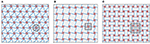Figure 1. Acoustic metamaterials characterized by periodic microstructure and different cell topologies: (A) hexachiral, (B) tetrachiral, (C) anti-tetrachiral.

FIGURE 2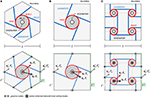Figure 2. Periodic microstructures and beam lattice models for different cell topologies: (A) hexachiral, (B) tetrachiral, (C) anti-tetrachiral.

A rigid body model is adopted for all the massive and highly-stiff rings, possessing mean diameter D. The small ring width S is considered a free parameter, allowing the independent assignment of the rigid body mass M and moment of rotational inertia J. A linear, extensible, unshearable model of massless beam is employed for all the light, flexible, and slender ligaments, in the small-deformation range. The beam-ring connections are ideally supposed to realize perfectly-rigid joints. By virtue of the geometric periodicity, the cell boundary usually crosses the midspan—and halves the natural length—of all the inter-cellular ligaments. Assuming the same linear elastic material (with Young's modulus E) and cross-section shape (with area A and second area moment I) for each ligament, all the beams have identical extensional rigidity EA and flexural rigidity EI. The effects of a homogeneous soft matrix, which may likely embed the microstructure, are neglected as first approximation.

Moving from this general microstructural layout, acoustic metamaterials can be realized by supplying each ring with a light soft annular filler, hosting a central heavy circular inclusion, serving as inertial resonator with adjustable mechanical properties. All the inclusions are modeled as rigid disks, co-centered with the respective housing rings, with body mass Mr and moment of rotational inertia Jr. As long as the internal (local) coupling provided by the filler can be assumed linearly elastic, the ring-resonator differential displacements are affected by equivalent translational and rotational stiffnesses (Bacigalupo and Gambarotta, 2016). Therefore, the local (translational and rotational) motion of each resonator is essentially characterized by its natural frequencies Ωr and Ωθ.

Introducing certain quantities as known dimensional references for the space (i.e., the characteristic length L of the periodic cell), a suited minimal-dimension vector μs of independent non-dimensional parameters, sufficient to describe the inertial, elastic and geometric properties of the periodic cell, can be introduced. Together with these microstructural parameters, the acoustic metamaterials are further characterized by a vector μr of additional non-dimensional parameters, describing the dynamic properties of the local resonators. Therefore, the vector μ = (μs, μr) can be defined to collect all the mechanical parameters. In the absence of resonators, it is understood that μ = μs.

### 2.1. Equations of Motion

According to the mechanical assumptions, the linear dynamics of the elementary cell is governed by a multi-degrees-of-freedom lagrangian model, referred to a set of N configurational nodes, pointed by the position vectors xi (with i = 1, …, N) in the natural configuration (see the lower part of Figure 2). The actual configuration of the i-th node is described by three time-dependent non-dimensional components of motion, corresponding to the horizontal displacement ui, the vertical displacement vi and the in-plane rotation ϕi. All the non-dimensional configuration variables can be collected in the 3N-by-one displacement column-vector q = (q1, …, qi, …, qN), where the i-th nodal subvector is qi = (ui, vi, ϕi).

Depending on the centroid position for the massive rings and the midspan position for the inter-cellular beams, the configurational nodes can conveniently be distinguished into three subsets:

i. Na internal nodes located at the massive ring centroids (internally to the elementary cell), whose 3Na active displacements can be collected in the subvector qa;

ii. Np external nodes located at the inter-cellular ligament midspans (lying exactly on the cell boundary), whose 3Np passive displacements can be collected in the subvector qp;

iii. Nr inner nodes located at the disk centroids, whose 3Nr active resonant displacements can be collected in the subvector qr.

The distinction remarks that the internal and inner nodes develop both elastic (σa, σr) and inertial forces (fa, fr), which actively participate in the dynamic cell equilibrium. On the contrary, the external nodes can develop only elastic forces σp, which quasi-statically balance the reactive forces fp transferred by the adjacent cells. Due to the geometric assumptions, the positions of the internal and inner node sets coincide in the undeformed configuration.

According to the partition of the displacement vector q = (qr, qa, qp) and partitioning the force vectors consistently, the non-dimensional equilibrium equation governing the undamped free oscillations of the discrete model has the matrix form

or, making explicit the force dependence on the nodal acceleration or displacements

where the dot indicates differentiation with respect to the non-dimensional time and O are matrices with all-zero entries.

Focusing on the micro-structural matrices, the global mass submatrix Ma is diagonal, as far as a lumped mass description is assumed. The symmetric submatrices Kaa and Kpp account for the global stiffness of the internal and external nodes, respectively. The rectangular submatrix ${\text{K}}_{ap}={\text{K}}_{pa}^{\top }$ expresses the elastic global coupling among the internal and external nodes. Focusing on the resonators, both the local mass and stiffness submatrices Mr and Kr are diagonal. The submatrix Kr accounts also for the global-local coupling between the inner and internal nodes.

### 2.2. Free Wave Propagation

The free wave propagation along the bi-dimensional cellular domain can be studied according to the Floquet-Bloch theory (Brillouin, 2003). In this respect, the active, resonant and passive displacement/force vectors can be mathematically related to their transformed counterparts ${\stackrel{~}{\text{q}}}_{a},{\stackrel{~}{\text{f}}}_{a},{\stackrel{~}{\text{q}}}_{r},{\stackrel{~}{\text{f}}}_{r},{\stackrel{~}{\text{q}}}_{p},{\stackrel{~}{\text{f}}}_{p}$ according to the Floquet-Bloch decomposition

where the block diagonal matrices Fa, Fr, Fp have j-th block (with I standing for the three-by-three unit matrix and j = 1, …, Na, j = 1, …, Nr, j = 1, …, Np respectively). In each block, i denotes the imaginary unit and k = (k1, k2) is the (dimensional) wavevector.

The cell boundary Γ can be separated into the complementary negative and positive sub-boundaries Γ and Γ+, where the external nodes are linked by the periodicity vectors d. Accordingly, the passive displacement and force vectors can be ordered and partitioned as ${\text{q}}_{p}=\left({\text{q}}_{p}^{-},{\text{q}}_{p}^{+}\right)$, ${\text{f}}_{p}=\left({\text{f}}_{p}^{-},{\text{f}}_{p}^{+}\right)$ to separate the variable pairs $\left({\text{q}}_{p}^{-},{\text{f}}_{p}^{-}\right)$ belonging to the negative sub-boundary Γ from the corresponding variable pairs $\left({\text{q}}_{p}^{+},{\text{f}}_{p}^{+}\right)$ belonging to the positive sub-boundary Γ+. Extending the same partition to the respective transformed variables, the Equation (3) can be written as

where, based on the decomposition, the block diagonal matrices ${\text{F}}_{p}^{-}$ and ${\text{F}}_{p}^{+}$ include the blocks related to the external nodes lying on the sub-boundaries Γ and Γ+, respectively.

Imposing the periodicity conditions on the transformed variables (${\stackrel{~}{\text{q}}}_{p}^{+}={\stackrel{~}{\text{q}}}_{p}^{-}$ and ${\stackrel{~}{\text{f}}}_{p}^{+}=-{\stackrel{~}{\text{f}}}_{p}^{-}$), the free wave propagation throughout the cell domain between the two complementary boundaries is governed by the quasi-periodicity conditions on the anti-transformed variables

where, following from the Equations (4), the block diagonal transfer matrix L has generic block where dij = xjxi represents the vector connecting the i-th external node (belonging to the sub-boundary Γ) and the j-th external node (belonging to the sub-boundary Γ+).

Consistently with the passive displacement and force decomposition, and imposing the quasi-periodicity conditions (5), the lower (quasi-static) part of Equation (2) reads

where I is a unit matrix of proper dimensions. This equation can be solved to express the passive variables as slave functions of the master active displacements, yielding

where the k-dependent auxiliary matrix $\text{R}=-\left(\text{L}{\text{K}}_{pp}^{\mp }\text{L}+\text{L}{\text{K}}_{pp}^{=}+{\text{K}}_{pp}^{#}\text{L}+{\text{K}}_{pp}^{±}{\right)}^{-1}$.

Similarly, the enforcement of the quasi-periodicity conditions to the upper (dynamic) part of the Equation (2) leads to a coupled equation that, after condensation of the passive variables by virtue of the enslaving relations (7), depends on the active variables only:

where the condensed stiffness matrix ${\text{K}}_{a}={\text{K}}_{aa}+\left({\text{K}}_{ap}^{-}+{\text{K}}_{ap}^{+}\text{L}\right)\text{R}\left({\text{K}}_{pa}^{+}+\text{L}{\text{K}}_{pa}^{-}\right)$ is known to be Hermitian by virtue of the symmetries ${\text{K}}_{ap}^{-}={\left({\text{K}}_{pa}^{-}\right)}^{\top }$ and ${\text{K}}_{ap}^{+}={\left({\text{K}}_{pa}^{+}\right)}^{\top }$.

As brief discussion, the upper part of Equation (8) governs the local dynamics of the resonator, whereas the lower part governs the global dynamics of the cell microstructure. It is worth noting that the passive variable condensation, including the enforcement of the quasi-periodicity, is not mathematically affected by the resonator presence. Indeed, the condensed global stiffness matrix Kg = (Kr + Ka) of the metamaterial is not formally different from the matrix Ka governing the wave propagation in the resonator-free material, apart for the mere addition of the local stiffness term Kr. Conversely, the uncoupled global dynamics of the resonator-free material can be restored by simply zeroing the local matrices Mr and Kr. Physically, this remark can immediately be justified by the absence of any internal coupling between the resonant active variables qr and the condensed passive variables qp.

Introducing the unknown non-dimensional frequency ω, the harmonic solutions and can be imposed in the Equation (8). Eliminating the dependence on time, an eigenproblem in the unknown eigenvalues λ = ω2 and eigenvectors ψ = (ψr, ψa) can be stated in the non-standard form (K − λM) = 0, or more explicitly

where the diagonal block matrices Fr = Fa have generic block $\text{I}{e}^{\text{i}\text{k}·{\text{x}}_{j}}$, where xj is the position vector pointing the active internal and inner nodes.

The eigenproblem solution gives Na + Nr real-valued eigenvalues λi (or frequencies ωi). It is worth remarking that, owing to the Hermitian property, the K-matrix is certainly non-defective, that is, possesses a complete eigenspace spanned by Na + Nr proper eigenvectors. Therefore, each eigenvalue λi has coincident algebraic and geometric multiplicity mi and corresponds to a complex-valued eigenvector ψi, collecting the sub-eigenvectors ψri and ψai. The passive sub-eigenvectors depend on the active sub-eigenvectors through the quasi-static relations ${\psi }_{pi}^{-}=\text{R}\left({\text{K}}_{pa}^{+}+\text{L}{\text{K}}_{pa}^{-}\right){\psi }_{ai}$ and ${\psi }_{pi}^{+}=\text{L}{\psi }_{pi}^{-}$.

Fixed an admissible vector μ of mechanical parameter values for the beam-lattice and the resonators, the eigenvalues (or frequencies) and the corresponding eigenvectors can be determined under variation of the non-dimensional wavevector b = (β1, β2), composed of the wavenumbers β1 = k1L and β2 = k2L ranging in the non-dimensional first Brillouin domain ${B}$ (Figure 3). The frequency loci vs. the varying wavevector constitute the Floquet-Bloch spectrum, composed of Na + Nr dispersion surfaces for the metamaterial or Na dispersion curves for the resonator-free material. In the spectrum, the two loci attaining zero value at the ${B}$-origin (β1 = β2 = 0) are referred to as acoustic surfaces. The other loci are referred to as optical surfaces.

FIGURE 3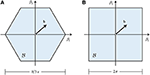Figure 3. Non-dimensional first Brillouin domain ${B}$ associated to: (A) hexachiral periodic cell, (B) tetrachiral and anti-tetrachiral periodic cell.

## 3. Band-Gap Optimization Problem

Parametric analyses can be performed by considering the dispersion functions ω(b, μ), where μ plays the role of multi-dimensional variable. These parametric analyses of the dispersion spectrum typically reveal that resonator-free materials possess a highly-dense spectrum, with persistent absence of full band-gaps in the low-frequency range (where only partial band gaps are usually obtainable). Introducing inter-ring resonators is an efficient technique to enforce the opening of stop bands in the closeness of the resonator frequency. Nonetheless, the resonator frequency cannot be reduced without recurring to high mass-to-stiffness ratio. Therefore, even in the presence of resonators, searching admissible parameter combinations that open low-frequency band-gaps—and hopefully maximize their bandwidth—is a challenging task, which can seldom be successfully approached manually. More efficient approaches can be represented by analytically inverting the eigenvalue problem, under acceptable asymptotic approximations (Lepidi and Bacigalupo, 2018b), or by numerically stating and solving optimization problems, under suited mechanical constraints (Bacigalupo et al., 2016a,b, 2017).

Within this context, the detection, quantification and—as a final target—design of metamaterials showing desiderable properties of mechanical filtering by virtue of low-frequency band-gap remains a research issue of major interest (D'Alessandro et al., 2016, 2018).

### 3.1. Problem Formulation

The bandwidth maximization of low-frequency band-gaps can be based on the definition of a suited μ-dependent objective function, which simultaneously accounts for the gap amplitude and the band center-frequency, hence qualifying in this respect as a multi-objective function. To this purpose, the following non-dimensional ratio can be defined:

where, supposing that the frequencies are sorted in ascending order, the numerator stands for the typically positive (even if possibly zero) gap amplitude between the k-th and h-th consecutive dispersion surfaces (where k = h + 1), while the denominator stands for the band center-frequency. When the numerator is negative, no band gap is present between the two surfaces.

Therefore, the optimization problem essentially consists in searching for the parameter vector μ that maximizes the objective function in the admissible parameter region. Consequently, the optimization issue can be mathematically formulated as a constrained maximization problem:

where μmin and μmax fix the boundaries of admissibility for the parameter vector, and g(μ) denotes a vector function defining additional relations, introduced—if necessary—to constrain a certain slave parameter as a known function of the other master parameters (Bacigalupo et al., 2016a, 2017). Altogether, the constraints define a properly-bounded space for the parameter vector.

Due to its mathematical formulation, the optimization problem turns out to be a challenging task in non-linear programming. Moreover, since the multi-variable objective function is not concave in the general case, the function maximization cannot be treated as a concave maximization problem. Multiple solutions associated to local maxima can co-exist. Therefore, the global maximum is necessarily approximated by the highest among several local maxima obtained numerically. If no full band gaps are found, then the optimization problem is re-formulated to search for partial band-gaps, by considering only certain directions of wave propagation.

Following a well-established methodology adopted for similar issues in material design (Sigmund and Jensen, 2003; Diaz et al., 2005), the optimization problem (11) can be solved by employing the Globally Convergent Method of Moving Asymptotes, or GCMMA (Svanberg, 1987, 2002). Loosely speaking, this solution method consists in tackling a sequence of concave-maximization subproblems, locally approximating the original non-linear optimization problem (a different approximation at each sequence iteration). In each subproblem, both the objective function and the constraints of the original optimization problem are approximated by separable functions, i.e., sums of functions depending each on a single different variable. This property simplifies solving the associated dual optimization subproblem. The moving asymptotes, which characterize the method, are asymptotes of the functions used in the approximations, and typically change from one subproblem to the successive one. Finally, the GCMMA method is globally convergent in the sense that, for every initial choice of the vector of optimization variables, it is proved to converge to a stationary point of the original optimization problem.

In Bacigalupo et al. (2016a,b, 2017), a quasi-Monte Carlo multi-start technique is also used in conjunction with the GCMMA, to increase the probability of finding a good approximation of the global maximum by virtue of a set of quasi-random initializations of the sequence. Indeed, the quasi-Monte Carlo initialization has the advantage—in comparison with the Monte Carlo initialization—of generating more uniform sequences of initial points (Figure 4). Furthermore, the quasi-Monte Carlo initialization, obtained at first generating a quasi-random Sobol' sequence (then keeping only elements of the sequence that satisfy the constraints of the original optimization problem), has the complementary advantage of being exactly replicable (Sobol, 1998).

FIGURE 4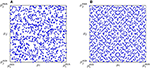Figure 4. Initialization of a 2-dimensional admissible region of the parameter domain: (A) Montecarlo sampling and (B) quasi-Montecarlo sampling.

For the sake of clarity, it is worth remarking that the present issue differs from other band gap maximization problems, which specifically deal with the topological optimization of phononic materials. Indeed, although pursuing the same objective (the largest gap amplitude), the topological optimization seeks for the optimal distribution of two or more material phases in a sufficiently-fine pixelation of the periodic cell (Cox and Dobson, 2000; Shen et al., 2003; Sigmund and Jensen, 2003; Kaminakis and Stavroulakis, 2012; Bruggi et al., 2017). On the contrary, here, for both the beam lattice material and metamaterial, the topology of the periodic cell is fixed a priori, whereas the parametric optimization is limited to the cellular micro-structural parameters, whose values allow to distinguish among different materials belonging to the same topological class. To some extent, the present analyses are aligned with the search for the maximum stop bandwidth achievable by varying the connection number and the joint rigidity in periodic lattices made of beam frameworks, in the absence of resonators (Wang et al., 2015).

## 4. Results and Discussion

The general mathematical form (11) of the optimization problem has been specialized for different chiral and anti-chiral topologies of materials and metamaterials. In particular, the maximal gap amplitude at the lowest center frequency has been searched for the hexachiral case (section 4.1), the tetrachiral case (section 4.2) and the anti-tetrachiral case (section 4.3).

### 4.1. Hexachiral Material and Metamaterial

The hexachiral material is characterized by a periodic cell with hexagonal shape and non-orthogonal periodicity vectors. Each cell contains one central ring connected to six tangent inter-cellular ligaments (Figure 2A). The beam lattice model is featured by three active degrees-of-freedom (Na = 3). The dispersion spectrum is composed by three frequency surfaces, defined over a hexagonal first Brillouin zone. The hexachiral metamaterial is realized by introducing a single local resonator in the central ring. Consequently, the beam lattice model is enriched by three active resonant degrees-of-freedom (Nr = 3). The corresponding dispersion spectrum is composed by three frequency surfaces in addition to those of the resonator-free material. The mass and stiffness matrices governing the eigenproblem (9) can be found in Bacigalupo and Gambarotta (2016) and Bacigalupo et al. (2016b).

The optimization problem for the hexachiral material can be formulated as the constrained maximization of a three-variables objective function defined according to Equation (10). Thus, the search for the optimal solution is performed in a properly-bounded three-dimensional space of the non-dimensional mechanical parameters expressing the ligament slenderness, the ring-to-cell aspect ratio and the chirality angle. The optimization problem is found not to admit solutions corresponding to full band gaps in the admissible parameter space. If the problem is reformulated to search for partial band-gaps, the largest stop bandwidth at the lowest center frequency is found between the second acoustic surface and the optical surface along the three propagation directions connecting two opposite-side vertices of the hexagonal cell (Bacigalupo et al., 2016b). The dispersion spectrum of the optimized hexachiral material is shown in Figure 5.

FIGURE 5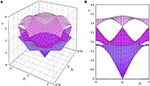Figure 5. Dispersion spectrum for the optimized beam lattice model of the hexachiral material: (A) 3D view and (B) 2D view.

The optimization problem for the hexachiral metamaterial can again be formulated as the constrained maximization of an enriched objective function, defined in an enlarged space of the non-dimensional mechanical parameters describing the hexachiral cell and the auxiliary resonator. The four extra non-dimensional parameters account for the resonator-to-cell aspect ratio, the resonator-to-ring mass ratio, and the two coefficients (Young modulus and Poisson ratio) of the elastic ring-to-resonator coupling. The optimization problem is found to admit a solution corresponding to a full band gap between the second acoustic surface and the optical surface (Bacigalupo et al., 2016b). The dispersion spectrum of the optimized hexachiral metamaterial is illustrated in Figure 6, where a second full band gap can be recognized to occur in the high-frequency range, between the fifth and sixth optical surfaces.

FIGURE 6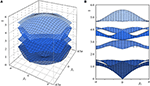Figure 6. Dispersion spectrum for the optimized beam lattice model of the hexachiral metamaterial: (A) 3D view and (B) 2D view.

From a qualitative viewpoint, it can be remarked that the largest amplitude of the full band gap can reach nearly half the maximum value of the lowest frequency in the spectrum of the hexachiral metamaterial. From a design perspective, it is worth pointing out that the optimized full band gaps can be obtained for small-radius rings and highly-slender, inclined but non-tangent ligaments. Correspondingly, the optimized resonators are found to possess nearly half the radius of the rings and be embedded in a highly-soft matrix.

### 4.2. Tetrachiral Material and Metamaterial

The tetrachiral material is characterized by a periodic cell with square shape and orthogonal periodicity vectors. Each cell contains one central ring connected to four tangent inter-cellular ligaments (Figure 2B). The beam lattice model is featured by three active degrees-of-freedom (Na = 3). The dispersion spectrum is composed by three frequency surfaces, defined over a square first Brillouin zone. The tetrachiral metamaterial is realized by introducing a single local resonator in the central ring. Consequently, the beam lattice model is enriched by three active resonant degrees-of-freedom (Nr = 3). The corresponding dispersion spectrum is composed by three frequency surfaces in addition to those of the resonator-free material. The mass and stiffness matrices governing the eigenproblem (9) can be found in Bacigalupo et al. (2016a) and Vadalà et al. (2018).

The optimization problem for the tetrachiral material can be formulated as the constrained maximization of a four-variables objective function defined according to Equation (10). Therefore, the search for the optimal solution is performed in a properly-bounded four-dimensional space of the non-dimensional mechanical parameters expressing the ligament slenderness, the ring-to-cell aspect ratio, the chirality angle and the ring-to-ligament width ratio. Similarly to the hexachiral case, the optimization problem for the tetrachiral material is found not to admit solutions corresponding to full band gaps in the admissible parameter space. If the problem is reformulated to search for partial band-gaps, the highest amplitude stop band at the lowest center frequency is found between the second acoustic surface and the optical surface along the two orthogonal propagation directions connecting the centroids of adjacent rings (Bacigalupo et al., 2016b). The dispersion spectrum of the optimized tetrachiral material is illustrated in Figure 7.

FIGURE 7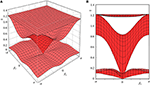Figure 7. Dispersion spectrum for the optimized beam lattice model of the tetrachiral material: (A) 3D view and (B) 2D view.

The optimization problem for the tetrachiral metamaterial can again be formulated as the constrained maximization of an enriched objective function, defined in the enlarged space of the non-dimensional mechanical parameters describing the tetrachiral cell and the auxiliary resonator. Similarly to the hexachiral case, the four extra non-dimensional parameters account for the resonator-to-cell aspect ratio, the resonator-to-ring mass ratio, and the two coefficients (Young modulus and Poisson ratio) of the elastic ring-to-resonator coupling. The constrained optimization problem is found not to have an admissible solution corresponding to a full band gap between the acoustic and optical surfaces. Instead, an admissible solution can be found for a full band gap separating the first and second optical surfaces. This solution preserves the ring-resonator interaction, by virtue of a weighting multiplier (proportional to the fourth pass bandwidth), properly applied to the stop bandwidth in the definition of the objective function (Bacigalupo et al., 2016a). The dispersion spectrum of the optimized tetrachiral metamaterial is illustrated in Figure 8.

FIGURE 8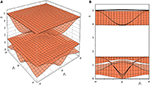Figure 8. Dispersion spectrum for the optimized beam lattice model of the tetrachiral metamaterial: (A) 3D view and (B) 2D view.

From a qualitative viewpoint, it can be remarked that the largest amplitude of the full band gap can be twice the maximum value of the lowest frequency in the spectrum of the tetrachiral metamaterial. From a design perspective, it is worth pointing out that the optimized full band gaps can be obtained for large-radius rings and highly-slender, non-tangent ligaments with quasi-negligible inclination (corresponding to a nearly-vanishing chirality angle). Similarly to the hexachiral case, the optimized resonators are found to possess nearly half the radius of the rings and be embedded in a highly-soft matrix.

### 4.3. Anti-tetrachiral Material and Metamaterial

The anti-tetrachiral material is characterized by a periodic cell with square shape and orthogonal periodicity vectors. Each cell contains four rings, each connected to four tangent (two inter-cellular and two intra-cellular) ligaments (Figure 2C). The beam lattice model is featured by 12 active degrees-of-freedom (Na = 12). The dispersion spectrum is composed by twelve frequency surfaces, defined over a square first Brillouin zone. The anti-tetrachiral metamaterial is realized by introducing a single local resonator in each ring. Consequently, the beam lattice model is enriched by twelve active resonant degrees-of-freedom (Nr = 12). The corresponding dispersion spectrum is composed by twelve frequency surfaces in addition to those of the resonator-free material. The mass and stiffness matrices governing the eigenproblem (9) can be found in Bacigalupo et al. (2017).

The optimization problem for the anti-tetrachiral material can be formulated as the constrained maximization of a three-variables objective function defined according to Equation (10). Since the chirality angle is not defined in the anti-chiral microstructural topology, the search for the optimal solution is performed in a properly-bounded three-dimensional space of the non-dimensional mechanical parameters expressing the ligament slenderness, the ring-to-cell aspect ratio and the ring-to-ligament width ratio. Similarly to the previous cases, the optimization problem for the anti-tetrachiral material is found not to admit solutions corresponding to full band gaps in the admissible parameter space. If the problem is reformulated to search for partial band-gaps, then the highest amplitude stop band at the lowest center frequency is found between the second and third optical surfaces along the two orthogonal propagation directions connecting diagonally two vertices of the square cell (Bacigalupo et al., 2017). The dispersion spectrum of the optimized anti-tetrachiral material is illustrated in Figure 9.

FIGURE 9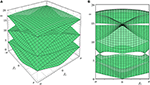Figure 9. Dispersion spectrum for the optimized beam lattice model of the anti-tetrachiral material: (A) 3D view and (B) 2D view.

The optimization problem for the anti-tetrachiral metamaterial can again be formulated as the constrained maximization of an enriched objective function, defined in the enlarged space of the non-dimensional mechanical parameters describing the tetrachiral cell and the auxiliary resonator. Similarly to the previous cases, the four extra non-dimensional parameters account for the resonator-to-cell aspect ratio, the resonator-to-ring mass ratio, and the two coefficients (Young modulus and Poisson ratio) of the elastic ring-to-resonator coupling. Furthermore, since the anti-tetrachiral material is characterized by a multi-ring cell, the number (ranging from one to four) and placement of the resonators can be considered additional unknowns to be optimized. The constrained optimization problem is found not to have an admissible solution corresponding to a full band gap between the acoustic and optical surfaces. Instead, an admissible solution can be found for a full band gap separating the fourth and fifth optical surfaces. This solution corresponds to two resonators placed into the ring pair located along one of the two diagonals of the square cell (Bacigalupo et al., 2017). It is worth noting that other solutions, maximizing the same objective function for a different number and placement of the resonators, correspond to full band gaps at higher center frequencies and lower stop bandwidth. The dispersion spectrum of the optimized anti-tetrachiral metamaterial is illustrated in Figure 10 (the lowest twelve dispersion surfaces are reported), where a second full band gap can be recognized to occur in the high-frequency range.

FIGURE 10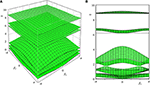Figure 10. Dispersion spectrum for the optimized beam lattice model of the anti-tetrachiral metamaterial: (A) 3D view and (B) 2D view.

From the qualitative viewpoint, the anti-tetrachiral metamaterial has been systematically found to offer the largest band gap amplitudes when the resonators are placed along one of the cell diagonals. From a design perspective, it is worth pointing out that the optimized full band gaps can be obtained by a strong ring-to-ligament compositeness of the cell microstructure (maximum admissible ring radius). Differently from the previous cases, the optimized resonators are found to be large and heavy (maximum admissible resonator inertia), but also weakly coupled with their hosting rings (minimum elastic resonator stiffness).

## 5. Band Design at Target Frequency

The numerical approaches to the spectral optimization can present two major applicative drawbacks. First, the admissible parameter space cannot overcome a certain dimension without compromising the computational feasibility (in terms of times and costs) of the optimal solution search. In this respect, different alternatives can turn out to be more convenient, if some simplifying hypotheses of the beam lattice model are relaxed (for instance, the lumped mass assumption). The consequent enlargement of the free parameter space offers more design possibilities, that can be explored by formulating continuous solid models. Their governing equations can be solved through numerical approaches (section 5.1). Second, the simplest numerical approaches to the optimization problem do not return an analytical form of the optimal solution (design parameters) as an explicit function of suitable objective variables. This shortcoming could be by-passed by analytically inverting and solving the spectral problem, provided that the designer accepts reasonable approximations. In this respect, perturbation-based techniques may offer a flexible mathematical tool to asymptotically approximate the inverse problem solutions (section 5.2).

### 5.1. Tetrachiral Material

Focusing on the reference lagrangian model for the tetrachiral material, a mechanical enrichment can be introduced by removing the strong hypothesis of massless ligaments. Furthermore, a heavy elastic material can be considered to fill the central rings of the periodic cell. According to these enrichments, the parameter space is enlarged by the ligament-to-ring mass ratio αb and the filler-to-ring mass ratio αi.

The admissible range of the enlarged parameter space—spanned by the additional mass ratios—has been investigated to check if some (αb, αi)-combinations realize a full band gap in the low-frequency pass band of the reference lagrangian model. The investigation results confirm that a low-frequency band gap with amplitude A and center frequency ϖ can be achieved. The achievable amplitude A vs. the mass ratio αb is shown in Figure 11A for different mass ratios αi. It is worth noting that the band gap amplitude A decreases monotonically for increasing values of the ligament-to-ring mass ratio αb. Larger amplitudes A can be obtained for higher filler-to-ring mass ratios αi. The center frequency ϖ shows a similar dependence on the mass ratio parameters, as illustrated is Figure 11B, where the pink zone embraces the frequency ranges included in the stop band for αi = 10. The figure could be used for design purposes by—for instance—fixing a target center frequency ϖ* (dashed line) to extract the corresponding design points (dots) in the αi and αb ranges. Further details can be found in Vadalà et al. (2018).

FIGURE 11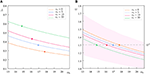Figure 11. Dispersion spectrum of the tetrachiral material: (A) full band gap amplitude A and (B) center frequency ϖ vs. the ligament-to-ring mass ratio αb for different filler-to-ring mass ratios αi.

### 5.2. Anti-tetrachiral Material

Focusing on the lagrangian models of beam lattice materials, a local sensitivity analysis of the dispersion spectrum can be performed by virtue of multi-parameter perturbation methods. This mathematical approach has been systematically formulated to achieve analytical—although asymptotically approximate up to the desired order—expressions for the dispersion relations, as explicit functions of the mechanical parameters and the wavenumbers. Within the limits of the local approximation, these analytical expressions have been found to closely fit the exact dispersion functions of the direct spectral problem for the anti-tetrachiral material, among the others.

The approximate analytical solutions of the direct spectral problem can properly be inverted to state convenient formulas to determine the design parameters as explicit functions of the inverse problem data. These target data may include—for instance—a desired frequency ϖ* at a certain wavenumber β* in the anti-tetrachiral material spectrum. Figure 12 shows how the fourth dispersion curve ω4(β) can be designed to include the desired frequencies ${\varpi }_{-}^{*}$ (slightly lower that unity - softer material) or ${\varpi }_{+}^{*}$ (slightly higher that unity - stiffer material) at different assigned wavenumbers β*. The design target pairs (ϖ*, β*) can be obtained for different anti-tetrachiral materials featured by low-density or high-density, to be selected according to extra design requirements. Further details can be found in Lepidi and Bacigalupo (2018b). Other achievable design targets are the stop bandwidth and the center frequency in the dispersion spectrum of anti-tetrachiral metamaterials (Lepidi and Bacigalupo, 2018a).

FIGURE 12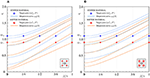Figure 12. Dispersion spectrum of the anti-tetrachiral material: dispersion curves ω4(β) designed to include the target frequencies ${\varpi }_{±}^{*}$ (for different wavenumbers β* = 0, 1/4π, 1/2π, 3/4π, π): (A) low-density material, (B) high-density material.

## 6. Conclusions and Future Developments

The dynamic response of composite materials with periodic microstructure can be analytically described by lagrangian beam lattice models. The free propagation of elastic waves in these low-dimensional models can be studied according to the Floquet-Bloch theory. The dispersion spectrum is composed of pass and stop bands that can be customized to let the material functionally behave as mechanical waveguide, filter or directional filter. Among the other functional features, low-frequency, high-amplitude band gaps are desirable spectral properties for many technological applications. To this purpose, the microstructural properties of the periodic cell can be employed as design parameters to optimize the band structure.

The optimization problem has been systematically formulated as a non-linear maximization problem by defining a non-concave multi-objective function, targeted at achieving the largest stop bandwidth at the lowest center frequency. The numerical search for the optimal solution has been opportunely constrained to focus on the admissible range of the design parameters. The optimization procedure can be applied to material and acoustic metamaterials governed by lagrangian models depending on a few mechanical parameters, including the number, placement and properties of local resonators.

Different cellular topologies have been optimized. Low-frequency high-amplitude full band gaps have been designed as outcome of the optimization process applied to hexachiral, tetrachiral and anti-tetrachiral metamaterials. According to the peculiar properties of microstructural symmetry assumed for the periodic cell, only partial band gaps for different propagation directions have been obtained in the absence of resonators. For each topology, design recommendations have been synthesized about the optimal inertial, elastic and geometric ratios among the microstructural parameters and the resonator properties.

As final achievements, alternative approaches to the spectral design have been summarized. First, the augmented design possibilities offered by an enlargement of the parameter space, resulting from the removal of some simplifying mechanical assumptions, have been explored. Second, the actual potential of perturbation methods to provide analytical, although asymptotically approximate, solutions of the inverse spectral problem has been successfully verified.

As outlook for future developments, the optimization strategy could be applied to the Rayleigh-Lamb wave propagation in heterogeneous macrostructures like multilayered beams or plates (Nayfeh et al., 1999; Chen et al., 2007; Pelassa and Massabò, 2015; Massabò, 2017). Furthermore, some shortcomings in the classical iterative algorithms for solving the optimization problem could be addressed. Specifically, a major improvement would be to reduce the time-consumption required to complete each iteration, depending on the model dimension, the wavevector discretization and the precision requirements. In this respect, a feasible countermeasure could be to replace the objective function with a more-easily computable approximation, exploiting techniques from surrogate optimization (Koziel and Leifsson, 2013). In this respect, the approximation can be based on a mesh-free method for interpolation, employing a finite number of strictly positive-definite Gaussian Radial Basis Functions (Fasshauer, 2007). According to this alternative, a two-phase procedure can be established, in which a suboptimal solution is, first, found via a ultra-fast global search based on the approximate objective (first phase) and, second, locally re-optimized using the original objective (second phase). Preliminary results have been obtained for the maximization of a specific partial band gap in the tetrachiral metamaterial (Bacigalupo and Gnecco, 2018). The findings are promising since, in each repetition, the values of the surrogate and original objective functions evolve in a similar way during the various iterations of the Sequential Linear Programming algorithm applied therein. Besides the reduction of the computational costs, a further advancement could be to merge parametric and topological optimization issues. Indeed, the techniques developed in this work could be applied also to topology optimization. A promising idea is the employment of level set methods (Sethian, 1999; Gibou et al., 2018) to represent the topology, since these methods allow for merging and splitting of contours during the optimization. The reduction of such a problem to a finite number of optimization parameters could be carried out by approximating the level set function by a neural network, perhaps composed by a single hidden layer and sigmoidal computational units (Haykin, 1994).

## Author Contributions

All authors listed have made a substantial, direct and intellectual contribution to the work, and approved it for publication.

## Conflict of Interest Statement

The authors declare that the research was conducted in the absence of any commercial or financial relationships that could be construed as a potential conflict of interest.

## Acknowledgments

We acknowledge financial support of the (MURST) Italian Department for University and Scientific and Technological Research in the framework of the research MIUR Prin15 project 2015LYYXA8, Multi-scale mechanical models for the design and optimization of micro-structured smart materials and metamaterials, coordinated by Prof. A. Corigliano. We also thankfully acknowledge financial support by National Group of Mathematical Physics (GNFMINdAM).

## References

Amendola, A., Krushynska, A., Daraio, C., Pugno, N. M., and Fraternali, F. (2018). Tuning frequency band gaps of tensegrity mass-spring chains with local and global prestress. Int. J. Solids Struct. 155, 47–56. doi: 10.1016/j.ijsolstr.2018.07.002

Bacigalupo, A., and Gambarotta, L. (2016). Simplified modelling of chiral lattice materials with local resonators. Int. J. Solids Struct. 83, 126–141. doi: 10.1016/j.ijsolstr.2016.01.005

Bacigalupo, A., and Gnecco, G. (2018). Metamaterial filter design via surrogate optimization. J. Phys. Conf. Ser. 1092:012043. doi: 10.1088/1742-6596/1092/1/012043

Bacigalupo, A., Gnecco, G., Lepidi, M., and Gambarotta, L. (2016a). “Design of acoustic metamaterials through nonlinear programming,” in Springer Lecture Notes in Computer Science - The Second International Workshop on Machine Learning, Optimization and Big Data - MOD 2016, eds G. Giuffrida, G. Nicosia, and P. Pardalos (Volterra: Springer), 170–181. doi: 10.1007/978-3-319-51469-7_14

Bacigalupo, A., Gnecco, G., Lepidi, M., and Gambarotta, L. (2017). Optimal design of low-frequency band gaps in anti-tetrachiral lattice meta-materials. Compos. B 115, 341–359. doi: 10.1016/j.compositesb.2016.09.062

Bacigalupo, A., and Lepidi, M. (2018). Acoustic wave polarization and energy flow in periodic beam lattice materials. Int. J. Solids Struct. 147, 183–203. doi: 10.1016/j.ijsolstr.2018.05.025

Bacigalupo, A., Lepidi, M., Gnecco, G., and Gambarotta, L. (2016b). Optimal design of auxetic hexachiral metamaterials with local resonators. Smart Mater. Struct. 25:054009. doi: 10.1088/0964-1726/25/5/054009

Bordiga, G., Cabras, L., Bigoni, D., and Piccolroaz, A. (in press). Free forced wave propagation in a rayleigh-beam grid: flat bands, dirac cones, vibration localization vs isotropization. Int. J. Solids Struct. doi: 10.1016/j.ijsolstr.2018.11.007

Brillouin, L. (2003). Wave Propagation in Periodic Structures: Electric Filters and Crystal Lattices. Mineola, NY: Courier Corporation.

Bruggi, M., Zega, V., and Corigliano, A. (2017). Synthesis of auxetic structures using optimization of compliant mechanisms and a micropolar material model. Struct. Multidiscipl. Opt 55, 1–12. doi: 10.1007/s00158-016-1589-9

Celli, P., and Gonella, S. (2014). Low-frequency spatial wave manipulation via phononic crystals with relaxed cell symmetry. J. Appl. Phys. 115:103502. doi: 10.1063/1.4867918

Chen, J., Pan, E., and Chen, H. (2007). Wave propagation in magneto-electro-elastic multilayered plates. Int. J. Solids Struct. 44, 1073–1085. doi: 10.1016/j.ijsolstr.2006.06.003

Colquitt, D., Jones, I., Movchan, N., and Movchan, A. (2011). Dispersion and localization of elastic waves in materials with microstructure. Proc. R. Soc. Lond. A 467, 2874–2895. doi: 10.1098/rspa.2011.0126

Cox, S. J., and Dobson, D. C. (2000). Band structure optimization of two-dimensional photonic crystals in h-polarization. J. Comput. Phys. 158, 214–224. doi: 10.1006/jcph.1999.6415

D'Alessandro, L., Belloni, E., Ardito, R., Corigliano, A., and Braghin, F. (2016). Modeling and experimental verification of an ultra-wide bandgap in 3d phononic crystal. Appl. Phys. Lett. 109:221907. doi: 10.1063/1.4971290

D'Alessandro, L., Zega, V., Ardito, R., and Corigliano, A. (2018). 3d auxetic single material periodic structure with ultra-wide tunable bandgap. Sci. Rep. 8:2262. doi: 10.1038/s41598-018-19963-1

Diaz, A., Haddow, A., and Ma, L. (2005). Design of band-gap grid structures. Struct. Multidiscipl. Opt. 29, 418–431. doi: 10.1007/s00158-004-0497-6

Fasshauer, G. E. (2007). Meshfree Approximation Methods with MATLAB, Vol. 6. London, UK: World Scientific. doi: 10.1142/6437

Gibou, F., Fedkiw, R., and Osher, S. (2018). A review of level-set methods and some recent applications. J. Comput. Phys. 353, 82–109. doi: 10.1016/j.jcp.2017.10.006

Haykin, S. (1994). Neural Networks: A Comprehensive Foundation. Prentice Hall PTR.

Huang, H., Sun, C., and Huang, G. (2009). On the negative effective mass density in acoustic metamaterials. Int. J. Eng. Sci. 47, 610–617. doi: 10.1016/j.ijengsci.2008.12.007

Kaminakis, N. T. and Stavroulakis, G. E. (2012). Topology optimization for compliant mechanisms, using evolutionary-hybrid algorithms and application to the design of auxetic materials. Comp. B Eng. 43, 2655–2668. doi: 10.1016/j.compositesb.2012.03.018

Koziel, S., and Leifsson, L. (2013). Surrogate-Based Modeling and Optimization: Applications in Engineering. New York, NY: Springer Science & Business Media.

Krushynska, A., Kouznetsova, V., and Geers, M. (2014). Towards optimal design of locally resonant acoustic metamaterials. J. Mech. Phys. Solids 71, 179–196. doi: 10.1016/j.jmps.2014.07.004

Krushynska, A., Miniaci, M., Bosia, F., and Pugno, N. (2017). Coupling local resonance with bragg band gaps in single-phase mechanical metamaterials. Extreme Mech. Lett. 12, 30–36. doi: 10.1016/j.eml.2016.10.004

Langley, R. (1994). On the modal density and energy flow characteristics of periodic structures. J. Sound Vibr. 172, 491–511. doi: 10.1006/jsvi.1994.1191

Langley, R. (1995). Wave transmission through one-dimensional near periodic structures: optimum and to random disorder. J. Sound Vibr. 188, 717–743. doi: 10.1006/jsvi.1995.0620

Langley, R. (1996). A transfer matrix analysis of the energetics of structural wave motion and harmonic vibration. Proc. R. Soc. Lond. A 452, 1631–1648. doi: 10.1098/rspa.1996.0087

Lepidi, M., and Bacigalupo, A. (2018a). Multi-parametric sensitivity analysis of the band structure for tetrachiral acoustic metamaterials. Int. J. Solids Struct. 136-137, 186–202. doi: 10.1016/j.ijsolstr.2017.12.014

Lepidi, M., and Bacigalupo, A. (2018b). Parametric design of the band structure for lattice materials. Meccanica 53, 613–628. doi: 10.1007/s11012-017-0644-y

Liu, Z., Chan, C. T., and Sheng, P. (2005). Analytic model of phononic crystals with local resonances. Phys. Rev. B 71:014103. doi: 10.1103/PhysRevB.71.014103

Liu, Z., Zhang, X., Mao, Y., Zhu, Y. Y., Yang, Z., Chan, C. T., and Sheng, P. (2000). Locally resonant sonic materials. Science 289, 1734–1736. doi: 10.1126/science.289.5485.1734

Martinsson, P., and Movchan, A. (2003). Vibrations of lattice structures and phononic band gaps. Q. J. Mech. Appl. Math. 56, 45–64. doi: 10.1093/qjmam/56.1.45

Massabò, R. (2017). Propagation of rayleigh-lamb waves in multilayered plates through a multiscale structural model. Int. J. Solids Struct. 124, 108–124. doi: 10.1016/j.ijsolstr.2017.06.020

Mei, J., Ma, G., Yang, M., Yang, Z., Wen, W., and Sheng, P. (2012). Dark acoustic metamaterials as super absorbers for low-frequency sound. Nat. Commun. 3:756. doi: 10.1038/ncomms1758

Nayfeh, A. H., Faidi, W., and Abdelrahman, W. (1999). An approximate model for wave propagation in piezoelectric materials. I. laminated composites. J. Appl. Phys. 85, 2337–2346. doi: 10.1063/1.369547

Paggi, M. (2010). Singular, hypersingular and singular free electromagnetic fields at wedge tips in metamaterials. Int. J. Solids Struct. 47, 2062–2069. doi: 10.1016/j.ijsolstr.2010.04.003

Pelassa, M., and Massabò, R. (2015). Explicit solutions for multi-layered wide plates and beams with perfect and imperfect bonding and delaminations under thermo-mechanical loading. Meccanica 50, 2497–2524. doi: 10.1007/s11012-015-0147-7

Phani, A. S., Woodhouse, J., and Fleck, N. (2006). Wave propagation in two-dimensional periodic lattices. J. Acous. Soc. Am. 119, 1995–2005. doi: 10.1121/1.2179748

Reda, H., Rahali, Y., Ganghoffer, J.-F., and Lakiss, H. (2016). Analysis of dispersive waves in repetitive lattices based on homogenized second-gradient continuum models. Comp. Struct. 152, 712–728. doi: 10.1016/j.compstruct.2016.05.080

Ronellenfitsch, H., Stoop, N., Forrow, A., and Dunkel, J. (2018). Designing spectral bandgaps in phononic networks. arXiv:1802.07214.

Ruzzene, M., Scarpa, F., and Soranna, F. (2003). Wave beaming effects in two-dimensional cellular structures. Smart Mater. Struct. 12, 363–372. doi: 10.1088/0964-1726/12/3/307

Sethian, J. A. (1999). Level Set Methods and Fast Marching Methods: Evolving Interfaces in Computational Geometry, Fluid Mechanics, Computer Vision, and Materials Science, Vol. 3. Cape Town: Cambridge University Press.

Shen, L., Ye, Z., and He, S. (2003). Design of two-dimensional photonic crystals with large absolute band gaps using a genetic algorithm. Phys. Rev. B 68:035109. doi: 10.1103/PhysRevB.68.035109

Sigmund, O. and Jensen, J. S. (2003). Systematic design of phononic band–gap materials and structures by topology optimization. Philos. Trans. R. Soc. Lond. A 361, 1001–1019. doi: 10.1098/rsta.2003.1177

Sobol, I. M. (1998). On quasi-monte carlo integrations. Math. Comput. Simul. 47, 103–112. doi: 10.1016/S0378-4754(98)00096-2

Spadoni, A., Ruzzene, M., Gonella, S., and Scarpa, F. (2009). Phononic properties of hexagonal chiral lattices. Wave Motion 46, 435–450. doi: 10.1016/j.wavemoti.2009.04.002

Svanberg, K. (1987). The method of moving asymptotes - a new method for structural optimization. Int. J. Num. Methods Eng. 24, 359–373. doi: 10.1002/nme.1620240207

Svanberg, K. (2002). A class of globally convergent optimization methods based on conservative convex separable approximations. SIAM J. Opt. 12, 555–573. doi: 10.1137/S1052623499362822

Tee, K., Spadoni, A., Scarpa, F., and Ruzzene, M. (2010). Wave propagation in auxetic tetrachiral honeycombs. J. Vibr. Acoust. 132, 031007. doi: 10.1115/1.4000785

Tie, B., Tian, B., and Aubry, D. (2013). Theoretical and numerical investigation of hf elastic wave propagation in two-dimensional periodic beam lattices. Acta Mech. Sin. 29, 783–798. doi: 10.1007/s10409-013-0087-1

Vadalà, F., Bacigalupo, A., Lepidi, M., and Gambarotta, L. (2018). Bloch wave filtering in tetrachiral materials via mechanical tuning. Comp. Struct. 201, 340–351. doi: 10.1016/j.compstruct.2018.05.117

Wang, P., Casadei, F., Kang, S. H., and Bertoldi, K. (2015). Locally resonant band gaps in periodic beam lattices by tuning connectivity. Phys. Rev. B 91:020103. doi: 10.1103/PhysRevB.91.020103

Wang, Y. F., Wang, Y. S., and Zhang, C. (2016). Two-dimensional locally resonant elastic metamaterials with chiral comb-like interlayers: Bandgap and simultaneously double negative properties. J. Acoust. Soc. Am. 139, 3311–3319. doi: 10.1121/1.4950766

Zhu, R., Huang, G., and Hu, G. (2012). Effective dynamic properties and multi-resonant design of acoustic metamaterials. J. Vibr. Acoust. 134:031006. doi: 10.1115/1.4005825

Keywords: architectured materials, wave propagation, dispersion properties, band gaps, local resonators, acoustic metamaterials

Citation: Bacigalupo A, Lepidi M, Gnecco G, Vadalà F and Gambarotta L (2019) Optimal Design of the Band Structure for Beam Lattice Metamaterials. Front. Mater. 6:2. doi: 10.3389/fmats.2019.00002

Received: 23 October 2018; Accepted: 07 January 2019;
Published: 31 January 2019.

Edited by:

Fernando Fraternali, University of Salerno, Italy

Reviewed by:

Georgios Theocharis, UMR6613 Laboratoire d'Acoustique de l'Universite du Maine (LAUM), France
Anastasiia O. Krushynska, Department of Civil, Environmental and Mechanical Engineering, University of Trento, Italy
Giuseppe Zurlo, National University of Ireland Galway, Ireland

Copyright © 2019 Bacigalupo, Lepidi, Gnecco, Vadalà and Gambarotta. This is an open-access article distributed under the terms of the Creative Commons Attribution License (CC BY). The use, distribution or reproduction in other forums is permitted, provided the original author(s) and the copyright owner(s) are credited and that the original publication in this journal is cited, in accordance with accepted academic practice. No use, distribution or reproduction is permitted which does not comply with these terms.

*Correspondence: Luigi Gambarotta, luigi.gambarotta@unige.it